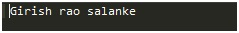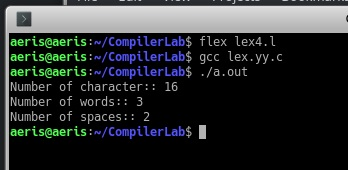# LEX Code to count and print the number of total characters, words, white spaces in given ‘Input.txt’ file

In this article, we going to learn how to create LEX program to analysis how many characters, white space (means \n \t “”), and words are present in given input file?
Submitted by Ashish Varshney, on March 24, 2018

For achieve to this task we create some rules in rule section in the code.

```/*Definition Section*/
%{
#include<stdio.h>
/*Global variables*/
int tchar=0,tword=0,tspace=0;
%}

/*Rule Section*/

%%

/*Increase the tspace and tword whenever
encounter whitespace.*/
" " {tspace++;tword++;}
/*Increase the tword whenever encounter newline
caharacter and tab mata character.*/
[\t\n] tword++;
[^\n\t] tchar++;

%%

/*call the yywrap function*/
int yywrap()
{
return 1;
}

int main()
{

yyin=fopen("q4.txt","r");
/*call the yylex function.*/
yylex();
printf("Number of character:: %d\nNumber of words::
%d\nNumber of spaces:: %d\n",tchar,tword,tspace);

return 0;
}
```

InputOutput